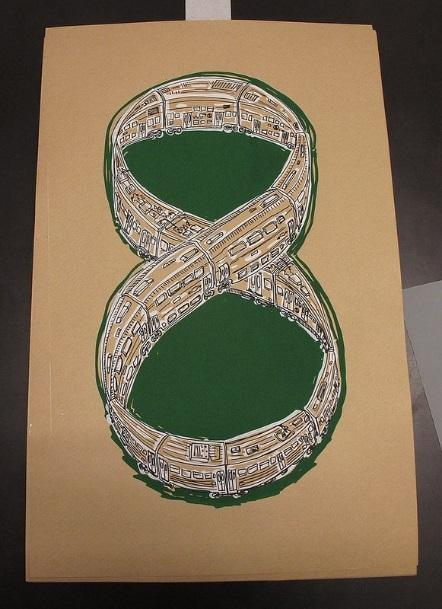# Read Useful Sample on How to Solve the Train Problem in Math

When you are having difficulties with math, sometimes it is not enough to study the theory. At times, you need to see how theoretical rules are applied in practice. You can find the examples of solutions to certain math problems on our blog. Below, you’ll find a sample on how to solve the train problem in math. This example was completed by an expert who is knowledgeable in math, as well as allied disciplines such as physics and engineering.

On AssignmentShark, you can find many samples on different technical disciplines which will help you to understand specific theoretical rules. Also, you can order a specific example completed just for you, requesting “do my math homework for me online” help from the top experts. Our experts work 24/7 so that students from different parts of the world can meet their deadlines and improve their scores. If you will need to know more on the issue than what is revealed in our example on how to solve the train problem in math, apply for our online assignment help as soon as possible. Remember that your deadline is looming closer by the minute!

## Infinite Train ProblemImagine a looped railroad. There we have a train, the last wagon of which is fastened to the first, so inside we can move freely between them. We are in a random wagon and our task is to count the total number of wagons. The trick is such that we can turn on or off the light in each wagon, but the initial position of the switches is random and we do not know it.

All the wagons inside look strictly the same, the windows are closed so it is impossible to see outside, and the movement of the train is uniform. We can’t mark the wagons in some different way, only activating or deactivating the light. The number of wagons is finite (don’t believe the title).

Here is one possible solution. We need to turn on the light in the initial wagon in which we are standing, if it is not yet lit. Then, we go in any direction until we encounter a wagon with working light, thus it is necessary to count the wagons. Turn off the light there and go back to the start. If the light is still lit, repeat the operation. If not, then we have gone full circle and know the answer.

With the same success it is possible, for example, to walk on the sides of the primary wagon at equal distances, gradually increasing them, and invert the light inside of them. That is, if we assume that first we are in the wagon with the number 0, then it is necessary to go in -1, 1, -2, 2, -3, 3, and so on. If we remember the state of the far wagon, when re-passing through it, we will notice a changed light if the circle closes. And knowing the length of the path in both directions, we can easily calculate the total number of wagons.